# Surface Area Lateral and Total Surface Area 8

• Slides: 33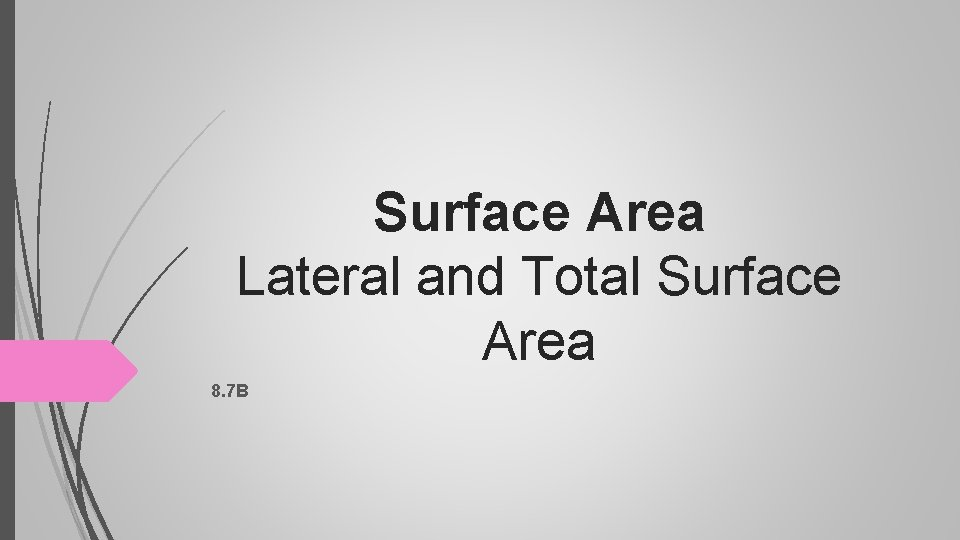Surface Area Lateral and Total Surface Area 8. 7 B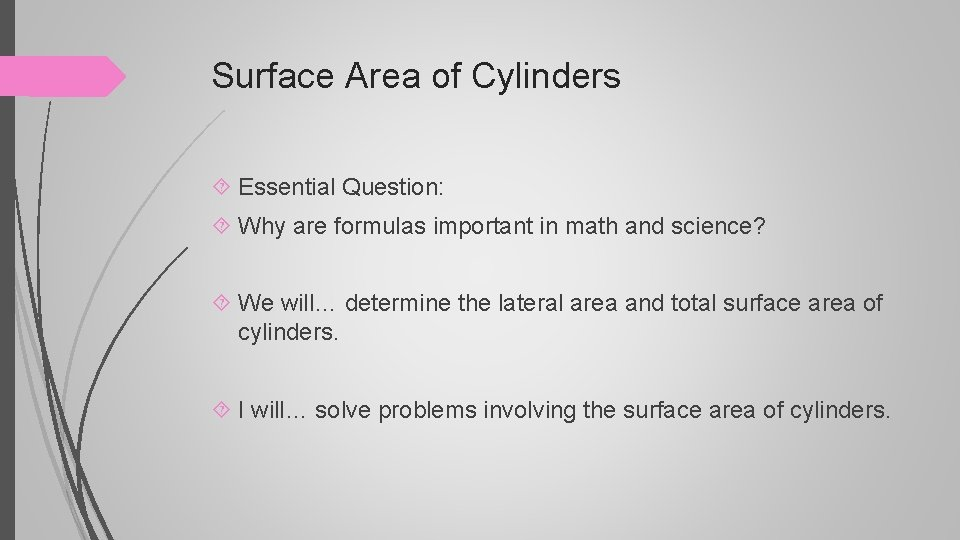Surface Area of Cylinders Essential Question: Why are formulas important in math and science? We will… determine the lateral area and total surface area of cylinders. I will… solve problems involving the surface area of cylinders.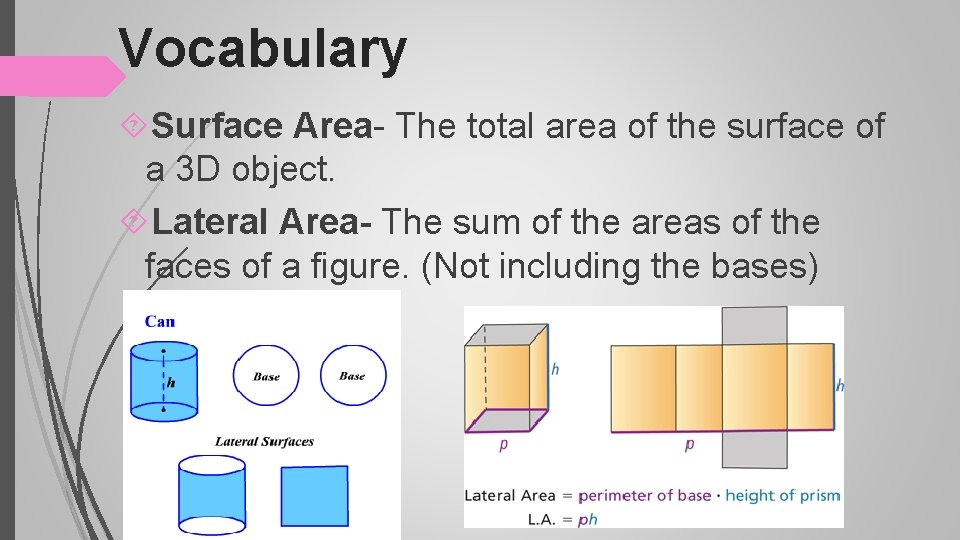Vocabulary Surface Area- The total area of the surface of a 3 D object. Lateral Area- The sum of the areas of the faces of a figure. (Not including the bases)Vocabulary Total Surface Area- The sum of the areas of ALL the faces including the bases.Lateral Surface area Formula:Total Surface area Formula: Lateral Formula Finding the area of BOTH bases.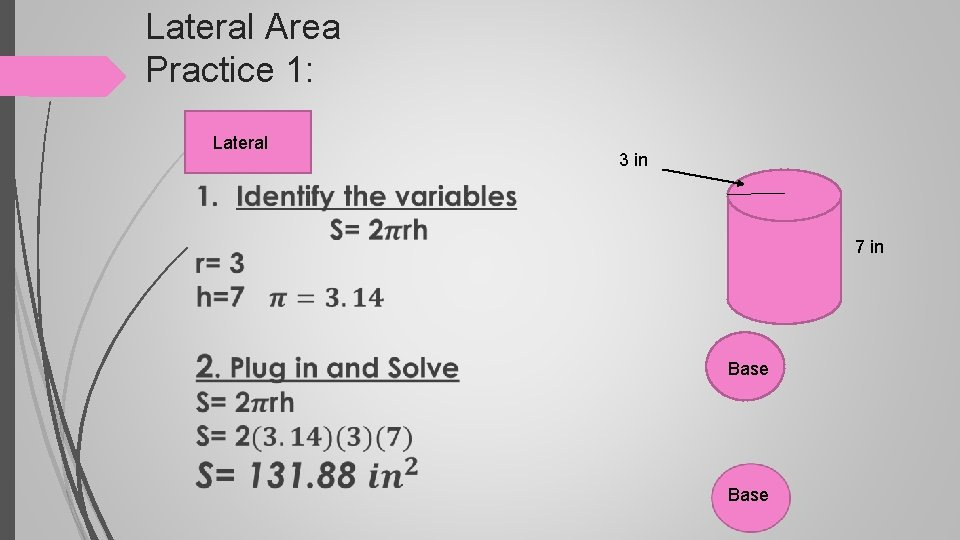Lateral Area Practice 1: Lateral 3 in 7 in BaseTotal Surface Area Base Lateral Formula Finding the area 3 in of BOTH bases. 7 in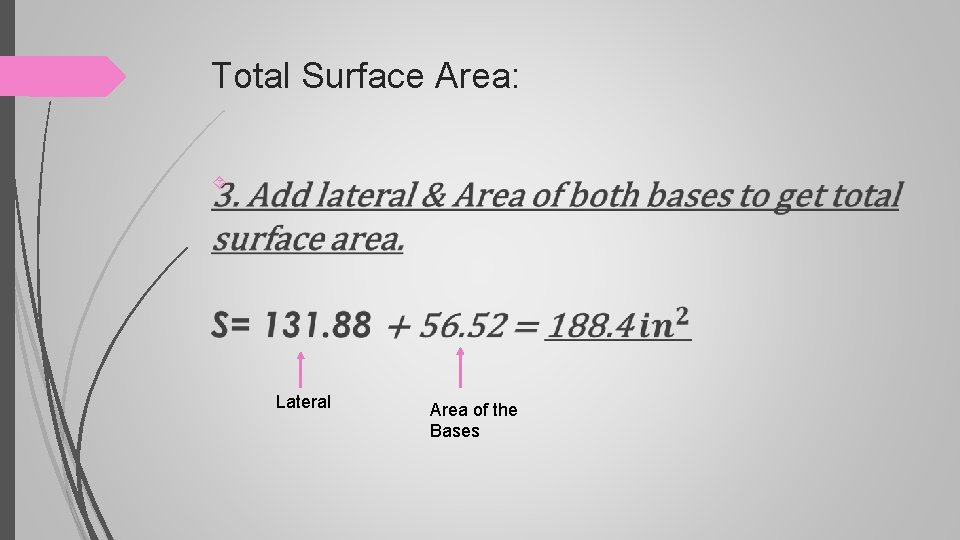Total Surface Area: Lateral Area of the Bases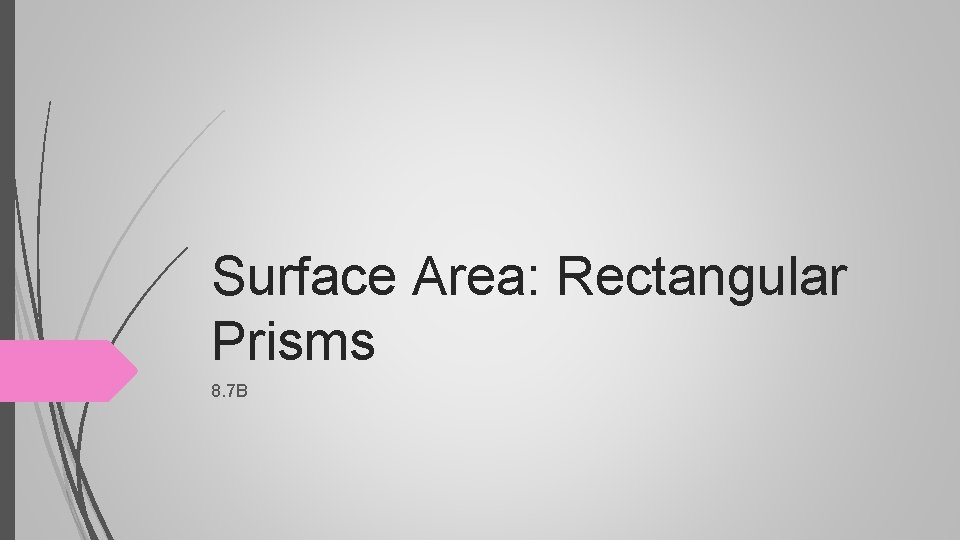Surface Area: Rectangular Prisms 8. 7 BWe will use previous knowledge of surface area to make connections to the formulas for lateral and total surface area. I will solve for lateral and surface area of a rectangular prism.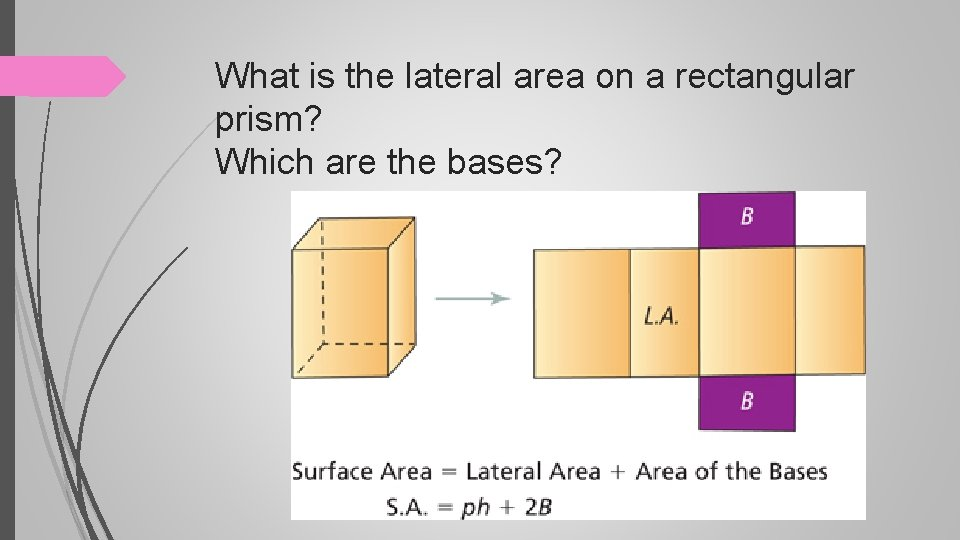What is the lateral area on a rectangular prism? Which are the bases?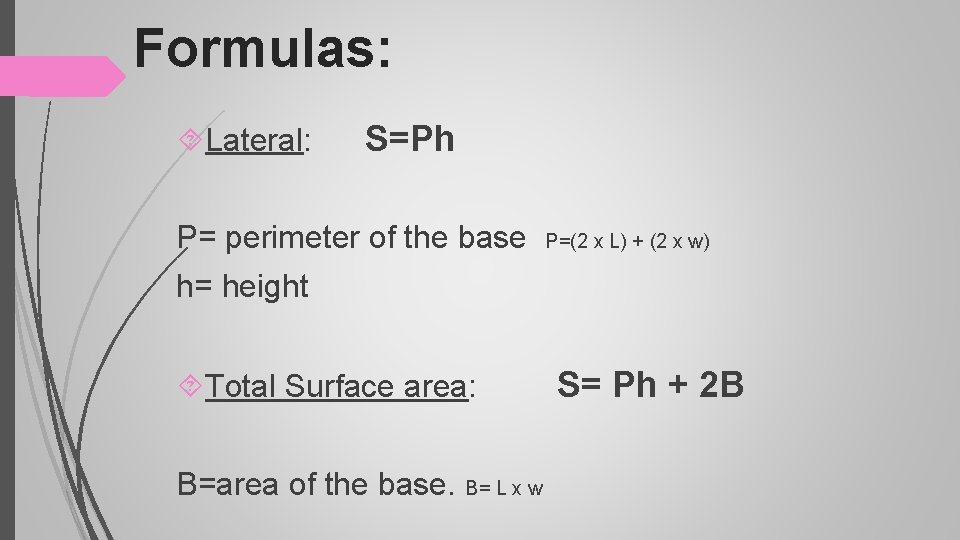Formulas: Lateral: S=Ph P= perimeter of the base P=(2 x L) + (2 x w) h= height Total Surface area: S= Ph + 2 B B=area of the base. B= L x w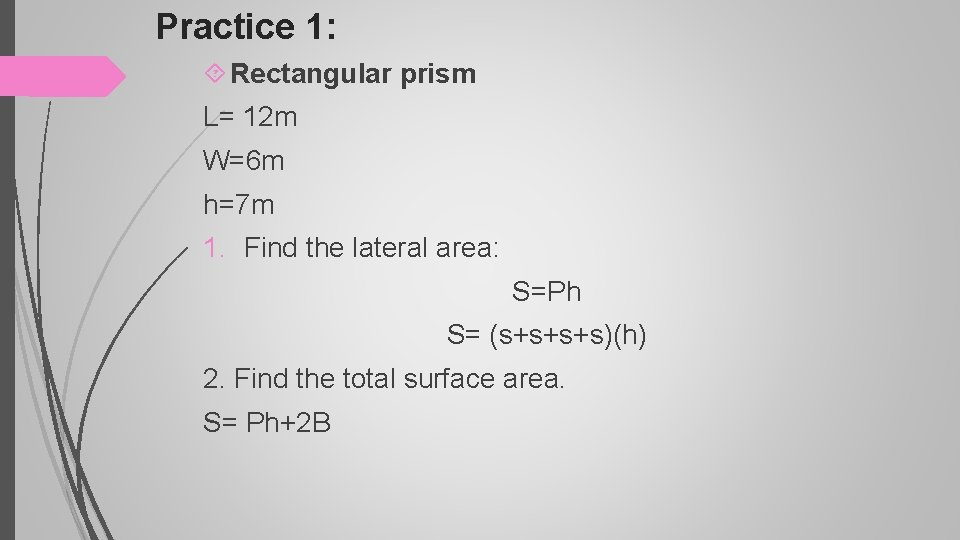Practice 1: Rectangular prism L= 12 m W=6 m h=7 m 1. Find the lateral area: S=Ph S= (s+s+s+s)(h) 2. Find the total surface area. S= Ph+2 BTotal Surface area: S= Ph + 2 BPractice 2: Rectangular prism L=14 ft W= 7 ft H=8 ft 1. Find the lateral area: S=Ph S= (s+s+s+s)(h) 2. Find the total surface area. S= Ph+2 BPractice 3: Rectangular prism L= 7 cm W= 3 cm H= 3 cm 1. Find the lateral area: S=Ph S= (s+s+s+s)(h) 2. Find the total surface area. S= Ph+2 BPractice 4: Rectangular prism L=4. 9 ft W= 3 ft H= 0. 7 1. Find the lateral area: S=Ph S= (s+s+s+s)(h) 2. Find the total surface area. S= Ph+2 B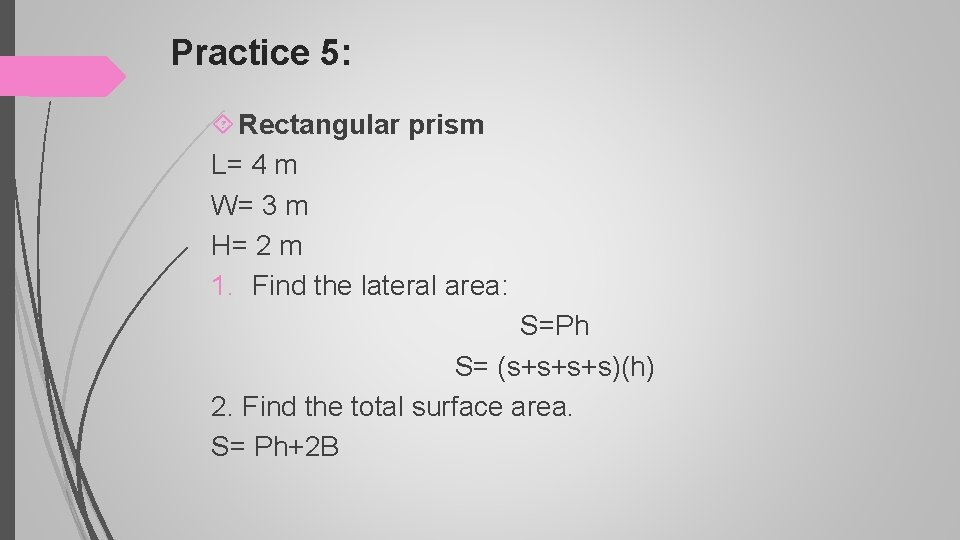Practice 5: Rectangular prism L= 4 m W= 3 m H= 2 m 1. Find the lateral area: S=Ph S= (s+s+s+s)(h) 2. Find the total surface area. S= Ph+2 B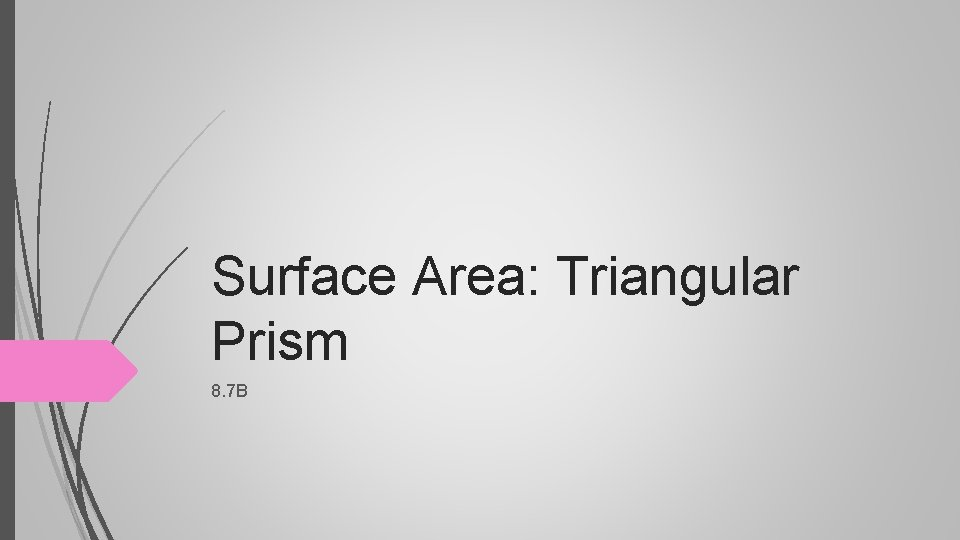Surface Area: Triangular Prism 8. 7 B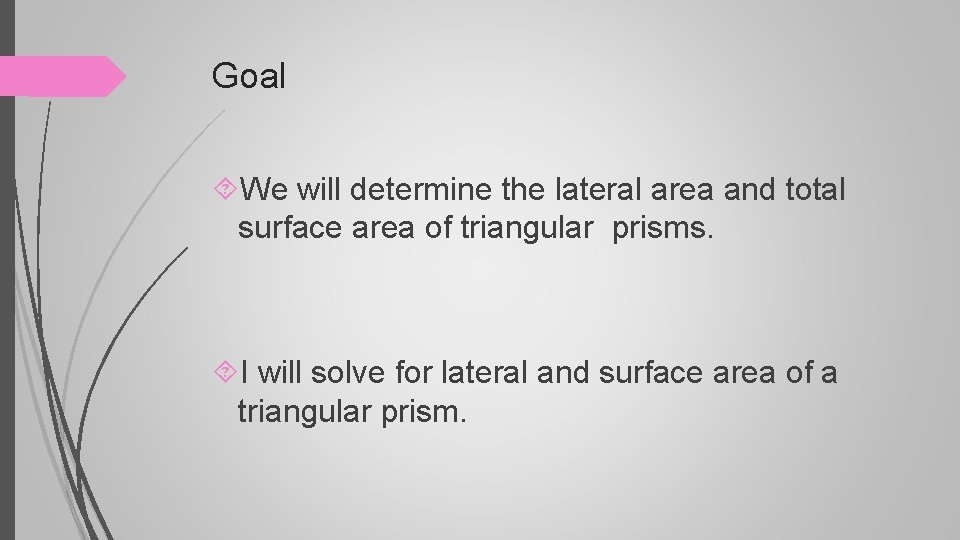Goal We will determine the lateral area and total surface area of triangular prisms. I will solve for lateral and surface area of a triangular prism.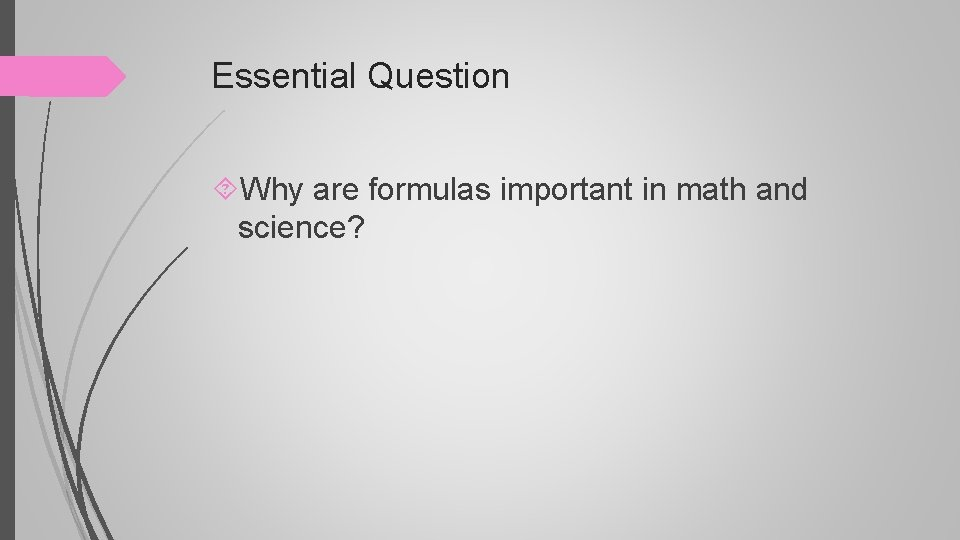Essential Question Why are formulas important in math and science?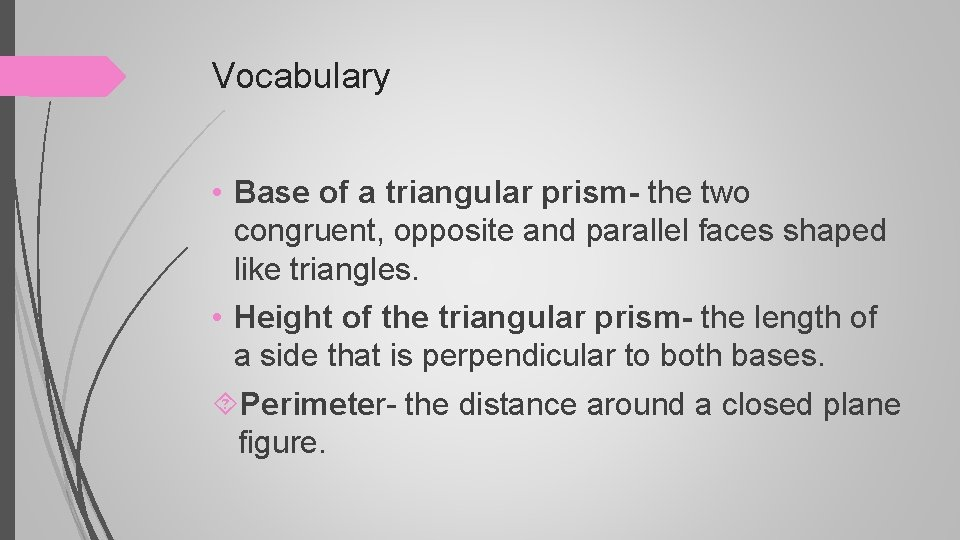Vocabulary • Base of a triangular prism- the two congruent, opposite and parallel faces shaped like triangles. • Height of the triangular prism- the length of a side that is perpendicular to both bases. Perimeter- the distance around a closed plane figure.Triangular Prism .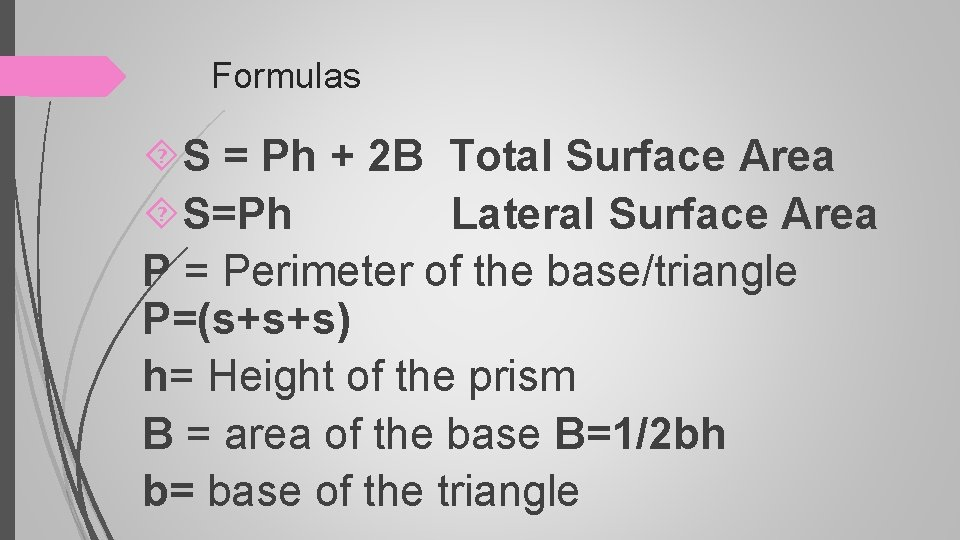Formulas S = Ph + 2 B Total Surface Area S=Ph Lateral Surface Area P = Perimeter of the base/triangle P=(s+s+s) h= Height of the prism B = area of the base B=1/2 bh b= base of the triangleTriangle Lateral Surface Area S= Ph 1. Solve for Lateral surface area. S= Ph h = 12 S= (s+s+s)(h) 13 18 15 S= (18+15+15)(13) S= 624 S = 624 ft square Dimensions Triangle: h= 12 b= 18 Rectangle: h=13 w= 15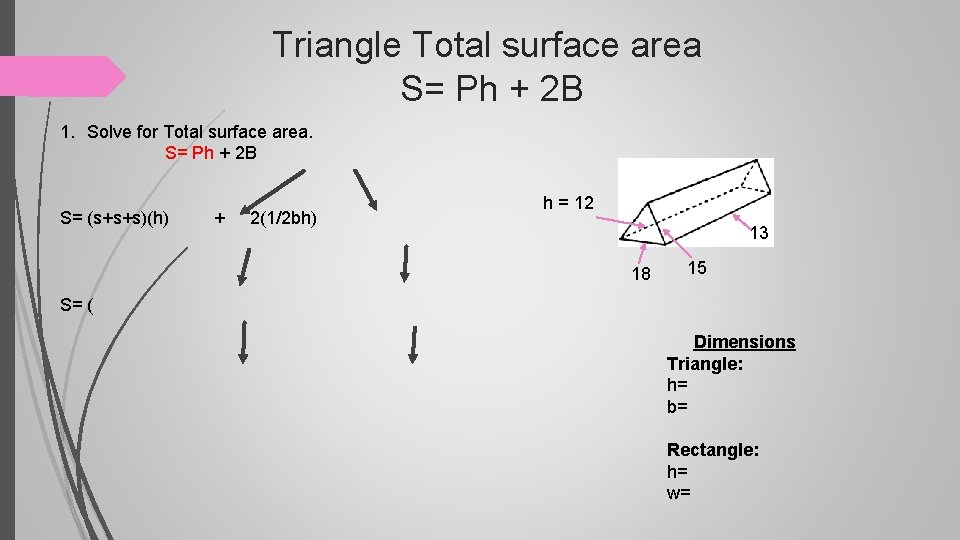Triangle Total surface area S= Ph + 2 B 1. Solve for Total surface area. S= Ph + 2 B S= (s+s+s)(h) + 2(1/2 bh) h = 12 13 18 15 S= ( Dimensions Triangle: h= b= Rectangle: h= w=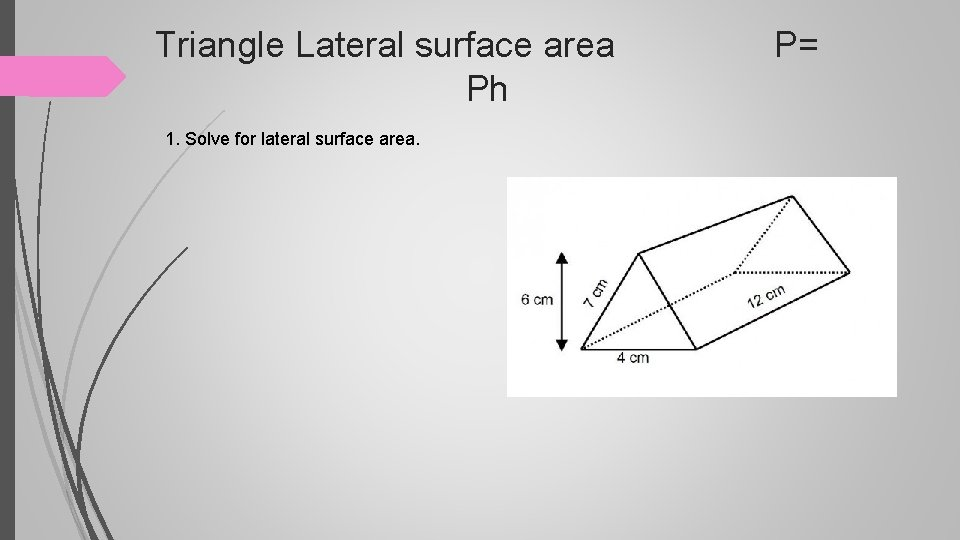Triangle Lateral surface area P= Ph 1. Solve for lateral surface area.Triangle Lateral surface area P= Ph + 2 B 1. Solve for lateral surface area.Triangle Lateral surface area P= Ph 1. Solve for lateral surface area.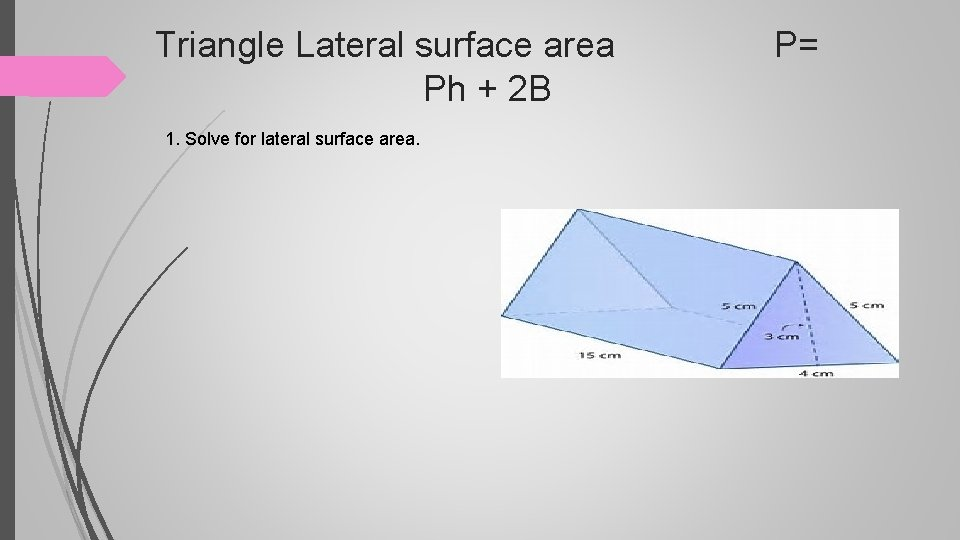Triangle Lateral surface area P= Ph + 2 B 1. Solve for lateral surface area.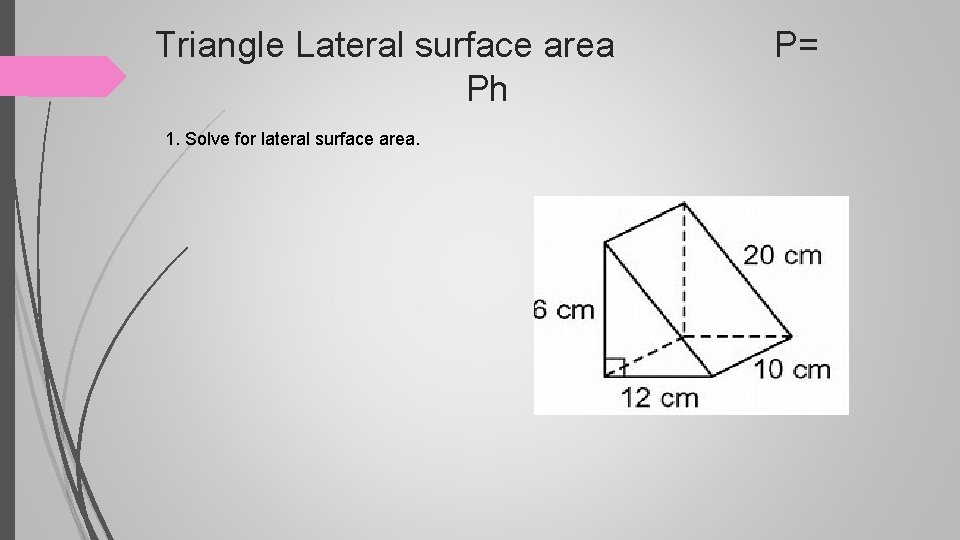Triangle Lateral surface area P= Ph 1. Solve for lateral surface area.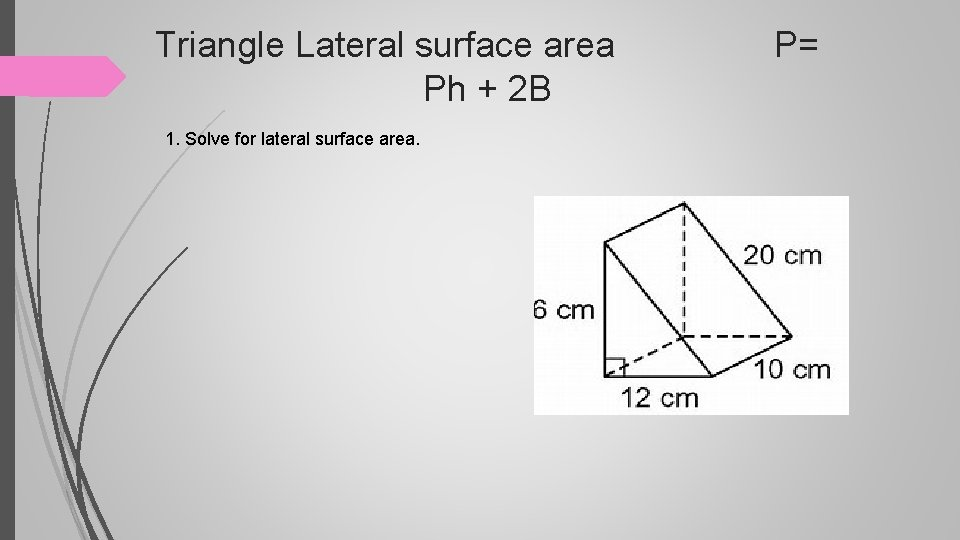Triangle Lateral surface area P= Ph + 2 B 1. Solve for lateral surface area.